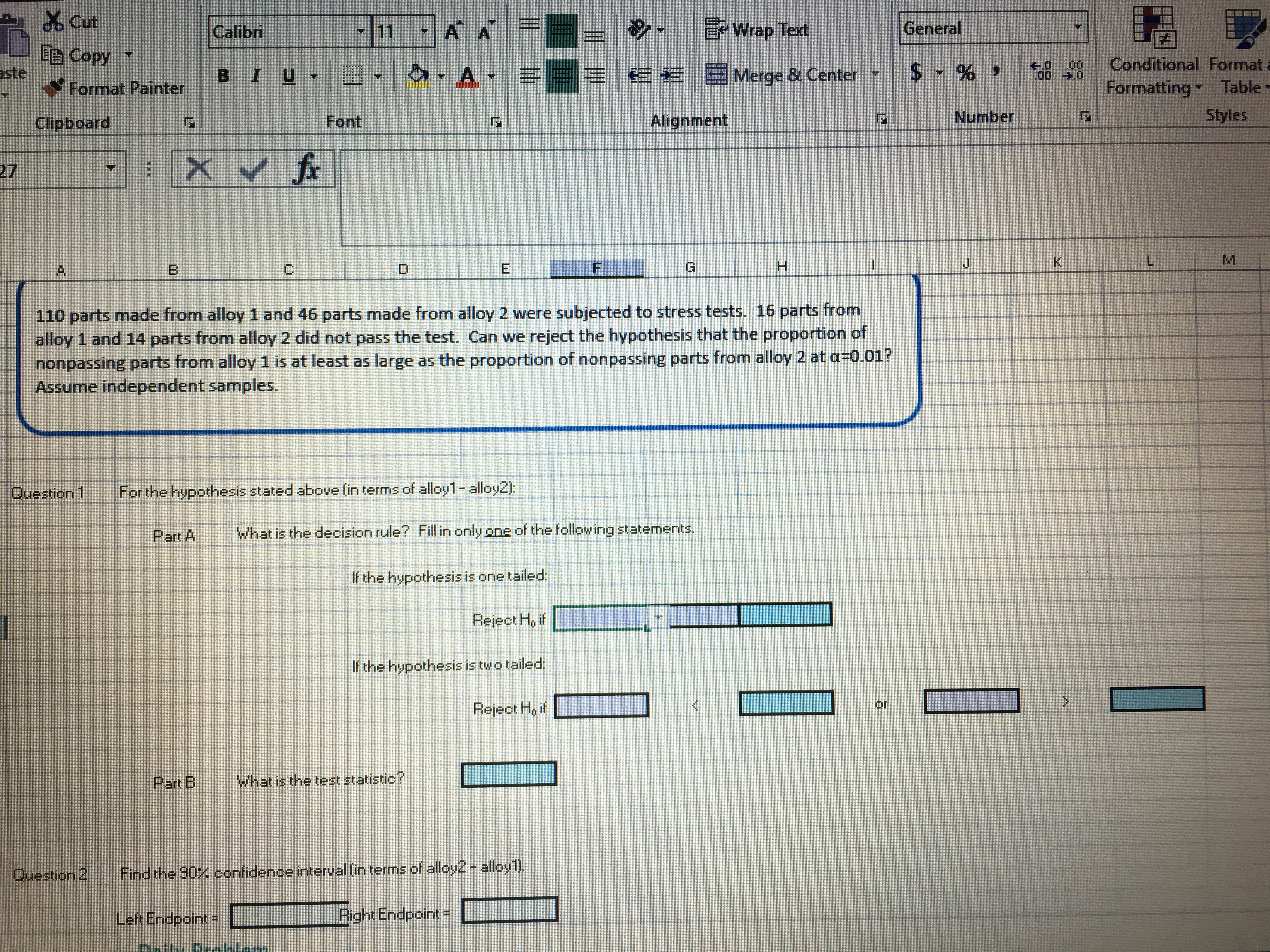# d Cut EE Copy ▼ H A 0 .00Conditional Format ste EEMerge & Center 00 a0 Format Painter Formatting- Table Number Clipboard Font Alignment Styles 27 Gi 110 parts made from alloy 1 and 46 parts made from alloy 2 were subjected to stress tests. 16 parts from alloy 1 and 14 parts from alloy 2 did not pass the test. Can we reject the hypothesis that the proportion of nonpassing parts from alloy 1 is at least as large as the proportion of nonpassing parts from alloy 2 at a-0.01? Assume independent samples Question 1 For the hypothesis stated above lin terms of alloy1- alloy2): Part A What is the decision rule? Fill in only one of the following statements. If the hypothesis is one tailed: If the hypothesis is twotailed: Reject Ho if nr What is the test statistic? 匚 Part B Question 2 Find the 90% confidence interval (in terms of alloy2-alloy!) Left Endpoint = Right Endpoint Ri

Questionhelp_outlineImage Transcriptionclosed Cut EE Copy ▼ H A 0 .00Conditional Format ste EEMerge & Center 00 a0 Format Painter Formatting- Table Number Clipboard Font Alignment Styles 27 Gi 110 parts made from alloy 1 and 46 parts made from alloy 2 were subjected to stress tests. 16 parts from alloy 1 and 14 parts from alloy 2 did not pass the test. Can we reject the hypothesis that the proportion of nonpassing parts from alloy 1 is at least as large as the proportion of nonpassing parts from alloy 2 at a-0.01? Assume independent samples Question 1 For the hypothesis stated above lin terms of alloy1- alloy2): Part A What is the decision rule? Fill in only one of the following statements. If the hypothesis is one tailed: If the hypothesis is twotailed: Reject Ho if nr What is the test statistic? 匚 Part B Question 2 Find the 90% confidence interval (in terms of alloy2-alloy!) Left Endpoint = Right Endpoint Ri fullscreen

### Want to see this answer and more?

Experts are waiting 24/7 to provide step-by-step solutions in as fast as 30 minutes!*